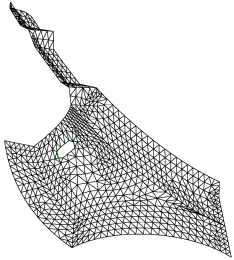# QTri

Applies to: Surfaces

Summary: Meshes surfaces using a quadrilateral scheme, then converts the quadrilateral elements into triangles.

Syntax:

Surface <range> Scheme Qtri [Base Scheme quad_scheme>]

QTri { Surface <range> | Face <range> }

Set QTri Split [2|4]

Set QTri Test {Angle|Diagonal}

Discussion:

QTri is used to mesh surfaces with triangular elements. The surface is, first, meshed with the quadrilateral scheme, and, then, the generated quads are split along a diagonal to produce triangles. The first command listed above sets the meshing scheme on a surface to QTri. The second form sets the scheme and generates the mesh in a single step.

In the first command, the user has the option of specifying the underlying quadrilateral meshing scheme using the base scheme <quad_scheme> option. If no base scheme is specified, CUBIT will automatically select a scheme. For non-periodic surfaces, the base scheme will be set to scheme pave. For periodic surfaces, the base scheme will be set to scheme map.

Generally, the second command, Qtri Surface <range>, is used on surfaces that have already been meshed with quadrilaterals. If, however, this command is used on a surface that has not been meshed, a base scheme will automatically be selected using CUBIT’s auto-scheme capabilities. The user can over-ride this selection by specifying a quadrilateral meshing scheme prior to using the qtri command (using the Surface <range> Scheme <quad_scheme> command). QTri may also be performed on quadrilateral elements on a surface or a subset of quadrilateral elements on a surface. To split existing quadrilaterals, the QTri command can be given a list of faces.

In addition to the default 2 tris per quad, the set qtri split command may alter the QTri scheme so that it will split the quad into 4 triangles per quad. Where the 4 option is used, an additional mesh node is placed at the centroid of each quad.

There are two methods that may be used to calculate the best diagonal to use for splitting the quadrilateral elements: angle or diagonal. The angle measurement uses the largest angle, while the diagonal option uses the shortest diagonal. The largest angle measurement will be more accurate but takes more time.

Also, the QTri scheme is used in the TriMesh command as a backup to the TriAdvance triangle meshing scheme.Figure 1. Surface meshed with scheme QTri